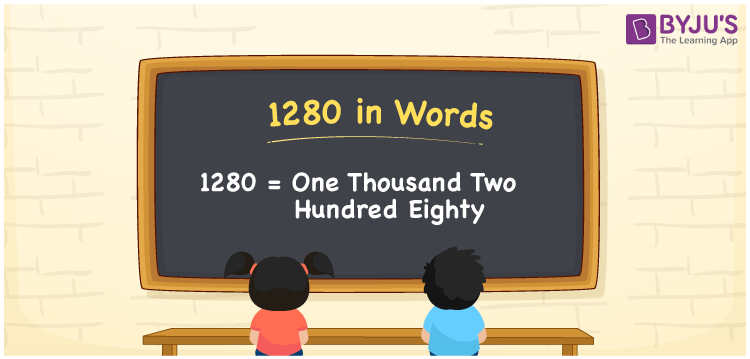# 1280 in Words

We can express 1280 in words as One Thousand Two Hundred Eighty. For example, Veena bought a pressure cooker worth Rs. 1280, and you can represent the same sentence in words as “Veena bought a pressure cooker worth Rupees One Thousand Two Hundred Eighty”. We know that 1280 is a cardinal number as it shows a certain amount. Let us learn how to convert the number 1280 into words using a place value chart with ease in this article.

 1280 in Words One Thousand Two Hundred Eighty One Thousand Two Hundred Eighty in numerical form 1280

## 1280 in English Words

Generally, numbers in words are represented using the English alphabet. Thus, we can write and read the number 1280 in English as One Thousand Two Hundred Eighty.## How to Write 1280 in Words?

Place value chart is important to write the numbers in word form. Here, let us make a chart of 4 columns to represent the place value chart for the number 1280 since it is a four-digit number.

 Thousands Hundreds Tens Ones 1 2 8 0

Therefore, we can write the expanded form as:

1 x Thousand + 2 x Hundred + 8 x Ten + 0 x One

= 1 x 1000 + 2 x 100 + 8 x 10 + 0 x 1

= 1000 + 200 + 80 + 0

= 1000 + 200 + 80

= 1280

= One Thousand Two Hundred Eighty

Hence, 1280 in words is written as One Thousand Two Hundred Eighty

Interesting way of writing 1280 in words

1 = One

12 = Twelve

128 = One Hundred and Twenty-Eight

1280 = One Thousand Two Hundred Eighty

Thus, the word form of the number 1280 is One Thousand Two Hundred Eighty

1280 is a natural number that precedes 1281 and succeeds 1279

• 1280 in words – One Thousand Two Hundred Eighty
• Is 1280 an odd number? – No
• Is 1280 an even number? – Yes
• Is 1280 a perfect square number? – No
• Is 1280 a perfect cube number? – No
• Is 1280 a prime number? – No
• Is 1280 a composite number? – Yes

## Frequently Asked Questions on 1280 in Words

Q1

### Write 1280 in words.

1280 in words is written as One Thousand Two Hundred Eighty.
Q2

### Simplify 1000 + 280, and express in words.

Simplifying 1000 + 280, we get 1280. Thus, 1280 in words is One Thousand Two Hundred Eighty.
Q3

### Write One Thousand Two Hundred Eighty in numbers.

One Thousand Two Hundred Eighty in numbers is 1280.
Test your Knowledge on 1280 in Words